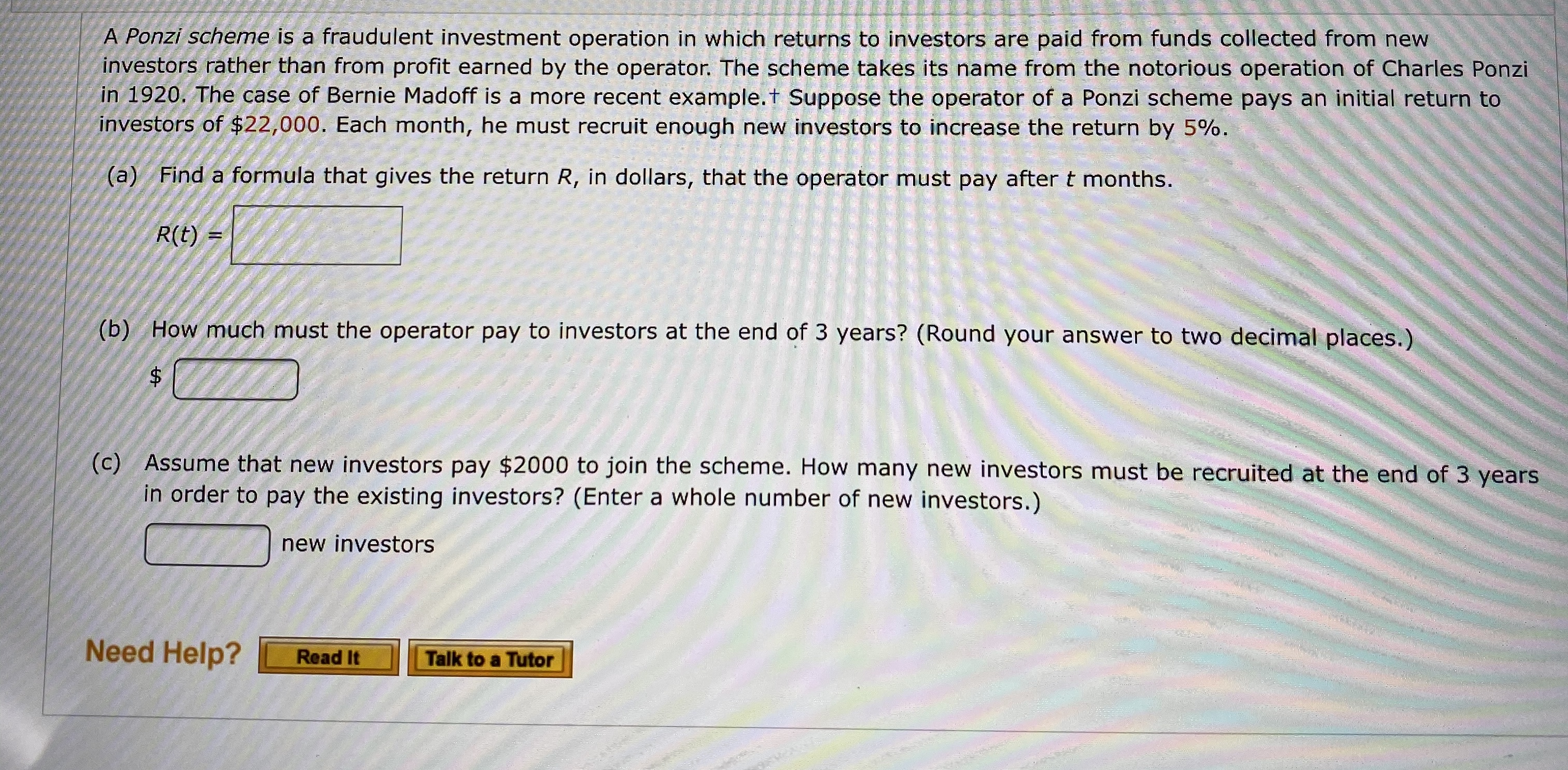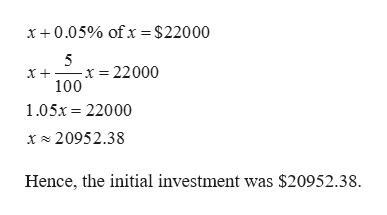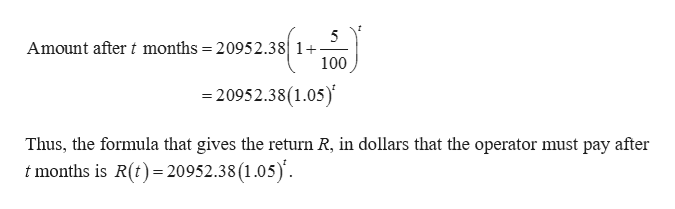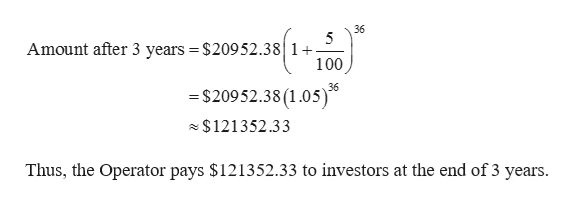# A Ponzi scheme is a fraudulent investment operation in which returns to investors are paid from funds collected from newinvestors rather than from profit earned by the operator. The scheme takes its name from the notorious operation of Charles Ponziin 1920. The case of Bernie Madoff is a more recent example.t Suppose the operator of a Ponzi scheme pays an initial return toinvestors of \$22,000. Each month, he must recruit enough new investors to increase the return by 5 % .Find a formula that gives the return R, in dollars, that the operator must pay after t months.(a)R(t)(b) How much must the operator pay to investors at the end of 3 years? (Round your answer to two decimal places.)\$(c) Assume that new investors pay \$2000 to join the scheme. How many new investors must be recruited at the end of 3 yearsin order to pay the existing investors? (Enter a whole number of new investors.)new investorsNeed Help?Read ItTalk to a Tutor

Question
15 views

What are the blanks?help_outlineImage TranscriptioncloseA Ponzi scheme is a fraudulent investment operation in which returns to investors are paid from funds collected from new investors rather than from profit earned by the operator. The scheme takes its name from the notorious operation of Charles Ponzi in 1920. The case of Bernie Madoff is a more recent example.t Suppose the operator of a Ponzi scheme pays an initial return to investors of \$22,000. Each month, he must recruit enough new investors to increase the return by 5 % . Find a formula that gives the return R, in dollars, that the operator must pay after t months. (a) R(t) (b) How much must the operator pay to investors at the end of 3 years? (Round your answer to two decimal places.) \$ (c) Assume that new investors pay \$2000 to join the scheme. How many new investors must be recruited at the end of 3 years in order to pay the existing investors? (Enter a whole number of new investors.) new investors Need Help? Read It Talk to a Tutor fullscreen
check_circle

Step 1

So if say x be the initial amount invested in the scheme then,help_outlineImage Transcriptionclosex+0.05% ofx \$2000 5 x = 22000 100 105x 22000 x20952.38 Hence, the initial investment was \$20952.38. fullscreen
Step 2

(a)

Since returns increase at a rate of 5% every month, use the formula for compound interest, with initial principal of \$20952.38; rate of interest at 5% and duration of investment as t months as follows,help_outlineImage Transcriptionclose5 Amount after t months = 20952.38 1 100 =20952.38(1.05) Thus, the formula that gives the return R, in dollars that the operator must pay after t months is R(t) 20952.38 (1.05 fullscreen
Step 3

(b)

3 years is same as 36 months so plug the value of t =36 in the above formula.

Thus the amount...help_outlineImage Transcriptionclose36 5 Amount after 3 years = \$20952.381+ 100 -\$20952.38(1.05) 36 \$121352.33 Thus, the Operator pays \$121352.33 to investors at the end of 3 years. fullscreen

### Want to see the full answer?

See Solution

#### Want to see this answer and more?

Solutions are written by subject experts who are available 24/7. Questions are typically answered within 1 hour.*

See Solution
*Response times may vary by subject and question.
Tagged in

### Other